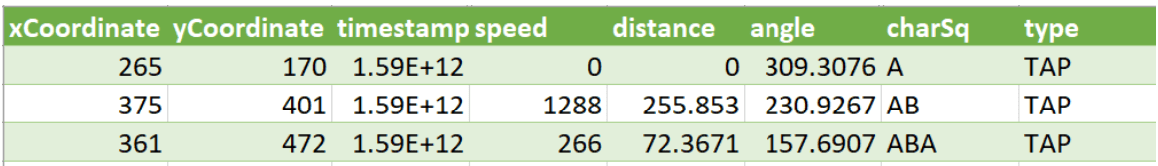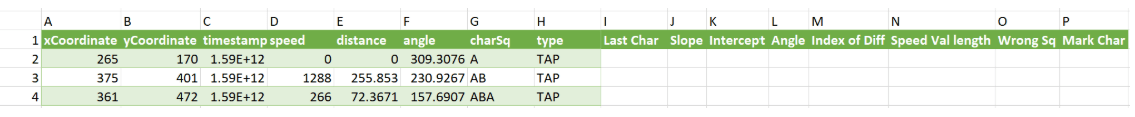# #StackBounty: #mysql #phpmyadmin #excel Multiple queries in phpmyadmin – Distance using coordinates, Slope, Intercept, Angle, and few m…

### Bounty: 50

I having around 500 excel sheets in .csv format with data captured for my experiment having following columns in place.Now I need to calculate the following parameters using this data. I have done these in excel, however doing this repeatedly for each excel so many times is difficult, so I want to write an SQL query in PhpmyAdmin will help some time.

1. Last charecter typed – need to capture last charecter from the column ‘CharSq’
2. *Slope (in column J) =(B3-B2)/(A3-A2)
3. Intercept (in column K) =B2-(A2*(J3))
4. Angle (in degrees) =MOD(DEGREES(ATAN2((A3-A2),(B3-B2))), 360) –
5. Index of Difficulty =LOG(((E1/7.1)+1),2)
6. Speed Value length (if speed value length >3, then mark as 1 or else 0) = =IF(LEN(D3) >= 3, "1","0")
7. Wrong Sequence (if I3=I2,then mark search time, else actual time) =IF(I3=I2,"Search Time","Actual Time")
8. Mark charecter into (1,2,3) = =IF(I2="A",1, IF(I2="B",2, IF(I2="C",3, 0)))I have started with this SQL query SELECT id, type, charSq, substr(charSq,-1,1) AS TypedChar, xCoordinate, yCoordinate, angle, distance, timestamp, speed FROM table 1 WHERE 1

Need help for the rest of the parameters. Thanks.

Note – I am going to run this in phpMyAdmin SQL

Get this bounty!!!

This site uses Akismet to reduce spam. Learn how your comment data is processed.